Next: Two ways to compute Up: CORRELATION AND SPECTRA Previous: CORRELATION AND SPECTRA

## Spectra in terms of Z-transforms

Let us look at spectra in terms of Z-transforms. Let a spectrum be denoted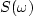, where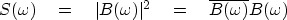(32)
Expressing this in terms of a three-point Z-transform, we have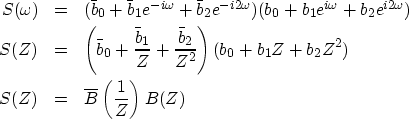(33) (34) (35)
It is interesting to multiply out the polynomial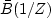with B(Z) in order to examine the coefficients of S(Z):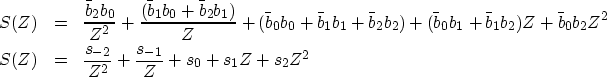(36)
The coefficient sk of Zk is given by(37)
Equation (37) is the autocorrelation formula. The autocorrelation value sk at lag 10 is s10. It is a measure of the similarity of bi with itself shifted 10 units in time. In the most frequently occurring case, bi is real; then, by inspection of (37), we see that the autocorrelation coefficients are real, and sk=s-k.

Specializing to a real time series gives(38) (39) (40) (41) (42)
This proves a classic theorem that for real-valued signals can be simply stated as follows:

 For any real signal, the cosine transform of the autocorrelation equals the magnitude squared of the Fourier transform.Next: Two ways to compute Up: CORRELATION AND SPECTRA Previous: CORRELATION AND SPECTRA
Stanford Exploration Project
10/21/1998